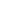| lesson material | material summary | questions and answers | definitions | types and examples | other information | materi pelajaran | ringkasan materi | pertanyaan dan jawaban | definisi | jenis-jenis dan contoh-contoh | informasi lainnya |

# Learning Javascript # 7: Understanding 6 Forms of Branching in JavascriptWhat is branching and why is it called branching?
For those who have never attended college or learned about algorithms and flowcharts, this might be the first term you hear.
Fill in this actually to describe the branching of the program flow.
In flow charts, the logic of "if ... then" is described in the form of a branch.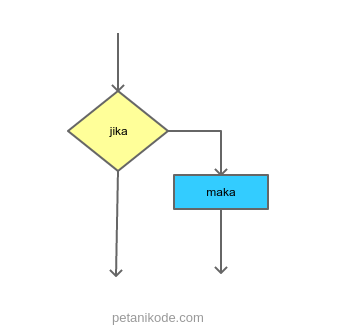Therefore, this is called branching.
In addition to branching, this structure is also called: flow control , decision , condition structure, if structure, etc.
Branching will be able to make the program think and determine actions according to the logic / conditions that we provide.

In Javascript programming, there are 6 forms of branching that we mustknow.
What are they?
Let's discuss ...

## 1. Branching if

Branching if the branching that only has one block selection when the condition is true.
Consider the following flowchart :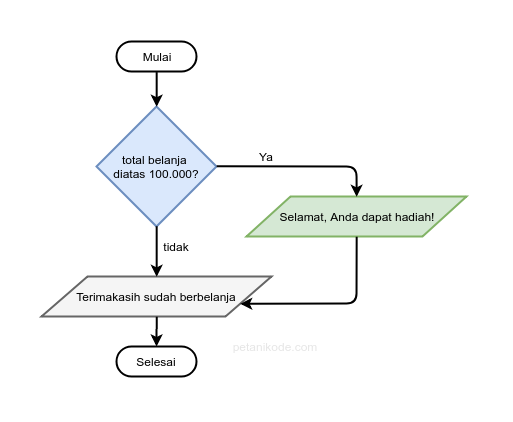We can read the flowchart like this:
"If the total expenditure is greater than IDR 100,000, then show the message Congratulations, you get a prize "
Yes the message is not displayed.
Let's try it in a Javascript program:
``````<!DOCTYPE html>
<html lang="en">
<title>Percabangan if</title>
<body>
<script>
var totalBelanja = prompt("Total belanja?", 0);

if(totalBelanja > 100000){
}

document.write("<p>Terimakasih sudah berbelanja di toko kami</p>");
</script>
</body>
</html>
``````
The result: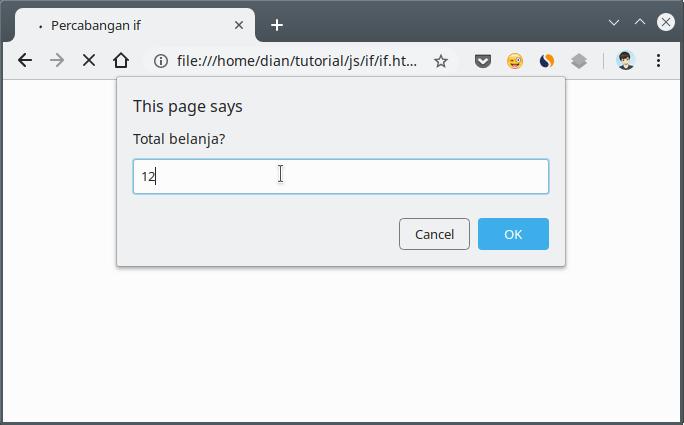Pay attention to this section:
``````if(totalBelanja > 100000){
}
``````
This is called a block.
The program block in Javascript, begins with an opening curly brackets `{`and ends with closing curly braces `}`.
If there is only one line of expression or statement in the block, then it may not be written in parentheses.
``````if(totalBelanja > 100000)
``````

## 2. If / else branching

An if / else branch is a branch that has two choice blocks .
The first choice for the condition completely , and the second choice for the conditions one ( else ).
Look at this flowchart: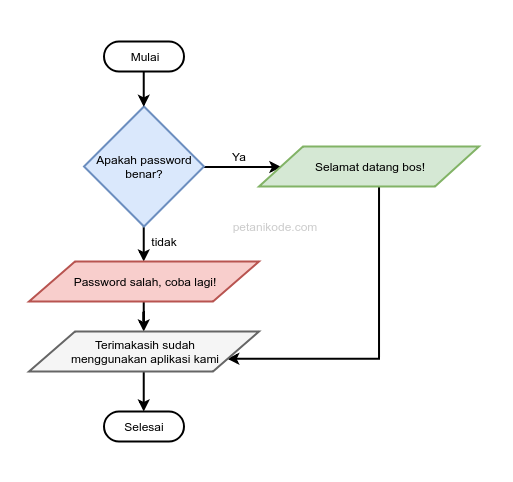This is a flowchart for checking passwords.
If the password is correct, a message on the green block will be displayed:"Welcome boss!"
But if it's wrong, then the message on the red block will be displayed: "The password is wrong, try again!"
Then, the message in the gray block will still be displayed, because it is not part of the if / else branching block .
Note the direction of the arrow, each if / else block leads to it ...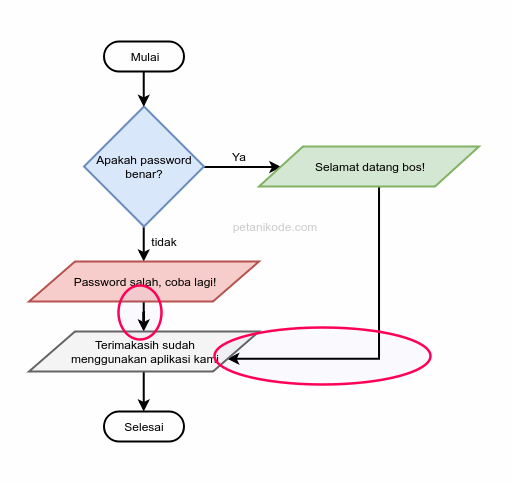For more details, let's try the program:
``````<!DOCTYPE html>
<html lang="en">
<title>Percabangan if/else</title>
<body>
<script>

document.write("<h2>Selamat datang bos!</h2>");
} else {
}

document.write("<p>Terima kasih sudah menggunakan aplikasi ini!</p>");

</script>
</body>
</html>
``````
The result: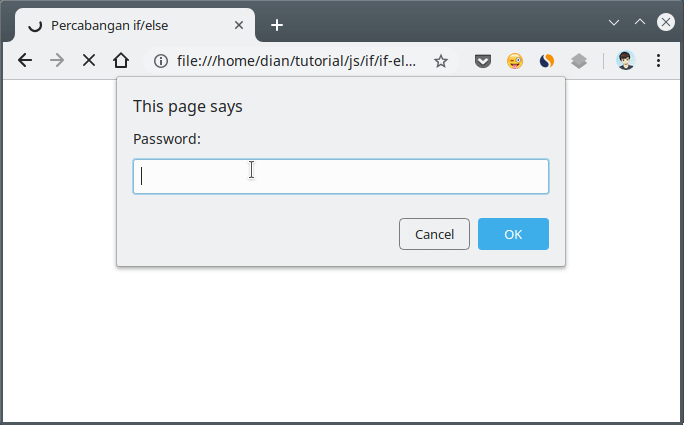## 3. If / else / if branching

Branching if / else / if a branch that has more than two blocks of choice .
Consider the following flowchart: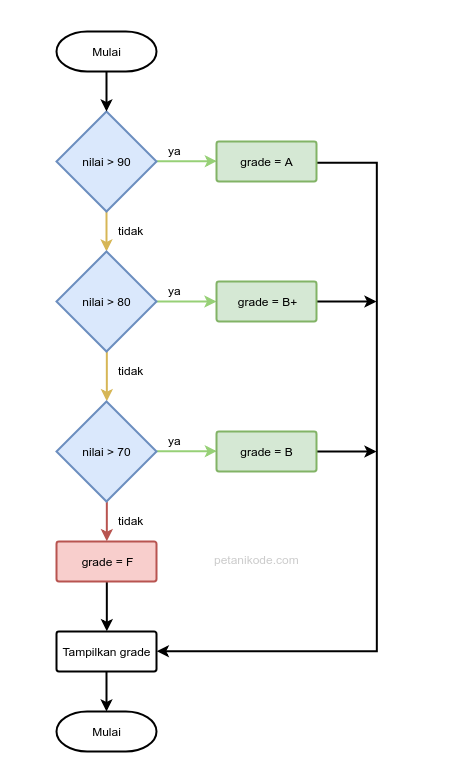Watch the block that I gave the color ...
This is a block for branching if / else / if . We can add whatever block we want.
Example Program:
``````<!DOCTYPE html>
<html lang="en">
<title>Percabangan if/else/if</title>
<body>
<script>
var nilai = prompt("Inputkan nilai akhir:");

if(nilai >= 90) grade = "A"
else if(nilai >= 80) grade = "B+"
else if(nilai >= 70) grade = "B"
else if(nilai >= 60) grade = "C+"
else if(nilai >= 50) grade = "C"
else if(nilai >= 40) grade = "D"
else if(nilai >= 30) grade = "E"

</script>
</body>
</html>
``````
The result: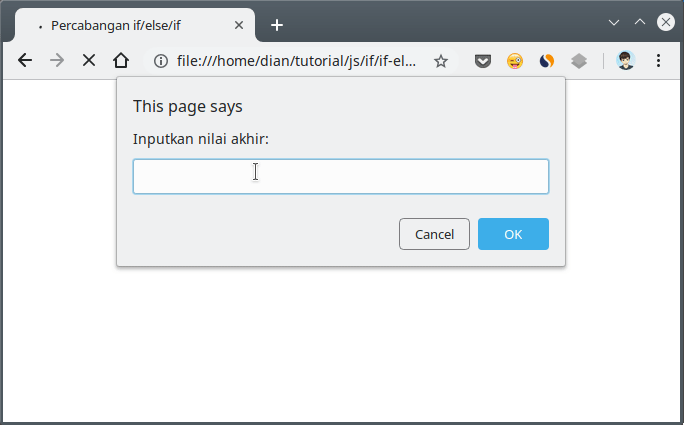In the above program, we do not use curly braces to block code for if / else / if.
because there is only one command line. Namely: `grade = ...`.
If we use curly braces, the program above will be like this:
``````<script>
var nilai = prompt("Inputkan nilai akhir:");

if (nilai >= 90){
} else if(nilai >= 80) {
} else if(nilai >= 70) {
} else if(nilai >= 60) {
} else if(nilai >= 50) {
} else if(nilai >= 40) {
} else if(nilai >= 30) {
} else {
}
</script>
``````

## 4. Branching / case branching

Switch / case branching is another form of if / else / if branching .
The structure is like this:
``````switch(variabel){
case <value>:
// blok kode
break;
case <value>:
// blok kode
break;
default:
// blok kode
}
``````
We can make `case`as many code blocks ( ) as desired in the switch block.
At `<value>`, we can fill in the values ​​that will be compared later `varabel`.
Each `case`must end with `break`Especially for `default`, it does not need to end`break`because he is located at the end.
The `break`purpose of the program is to stop the program from checking `case`next when it is `case` fulfilled.
Example:
``````<!DOCTYPE html>
<html lang="en">
<title>Percabangan switch/case</title>
<body>
<script>

var jawab = prompt("Kamu beruntung! Silahakn pilih hadiahmu dengan memasukan angka 1 sampai 5");

switch(jawab){
case "1":
break;
case "2":
break;
case "3":
break;
case "4":
break;
case "5":
break;
default:
document.write("<p>Opps! anda salah pilih</p>");
}

} else {
document.write("<h2>Selamat kamu mendapatkan " + hadiah + "</h2>");
}
</script>
</body>
</html>
``````
The result: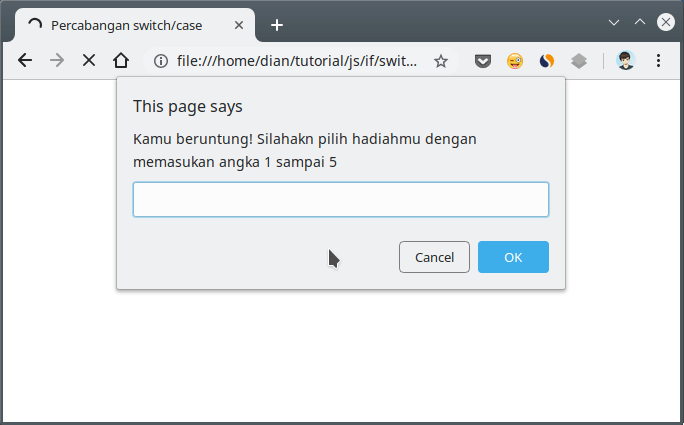Switch / case branching can also be made like this:
``````var nilai = prompt("input nilai");

switch(true){
case nilai < 90:
break;
case nilai < 80:
break;
case nilai < 70:
break;
case nilai < 60:
break;
case nilai < 50:
break;
case nilai < 40:
break;
case nilai < 30:
break;
default:
}
``````
First, we give a value `true`to `switch`, so we can get into the block `switch`.
Then inside the block `switch`, we create conditions using `case`.
The result will be the same as in the example of if / else / if branching .

## 5. Branching with Ternary Operators

Branching using ternary protectors is another form of if / else branching .
It can be said that:
The short form of if / else .
Example:
``````<!DOCTYPE html>
<html lang="en">
<title>Percabangan Ternary</title>
<body>
<script>
var jwb = prompt("Apakah Jakarta ibu kota indonesia?");

var jawaban = (jwb.toUpperCase() == "IYA") ? "Benar": "Salah";

document.write(`Jawaban anda: <b>\${jawaban}</b>`);
</script>
</body>
</html>
``````
The function of the method is `toUpperCase()`to convert the entered text to all capital letters.
The result: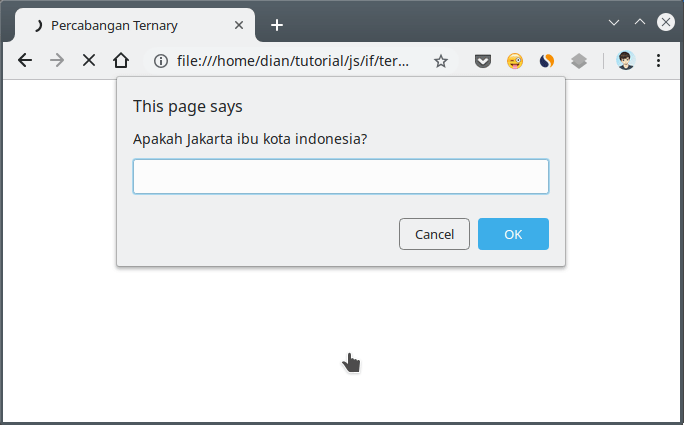The ternary Opertor acts as an if / else branch :
``````var jawaban = (jwb.toUpperCase() == "IYA") ? "Benar": "Salah";
``````
If the conditions in parenthesis - - `(jwb.toUpperCase() == "IYA")`value `true`, then the contents of the variable `jawaban`will be the same as `"Benar"`.
But if it's worth `false`, then the variable `jawaban`will contain `"Salah"`.

## 6. Branching Nesting (Nested)

We can also make branching blocks in branching. This is called a nested ifbranched branch .
Example:
``````<!DOCTYPE html>
<html lang="en">
<title>Percabangan Ternary</title>
<body>
<script>

document.write("<h2>Selamat datang pak bos!</h2>");
} else {
}
} else {
document.write("<p>Anda tidak terdaftar!</p>");
}
</script>
</body>
</html>
``````
The result: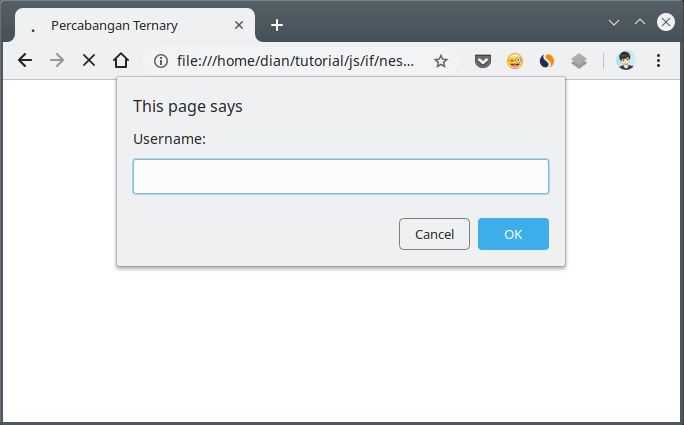## Bonus: Using Logic Operators in Branching

Branching is nested, we can actually make it even simpler by using a logical operator.
Example:
``````var username = prompt("Username:");

document.write("<h2>Selamat datang pak bos!</h2>");
} else {
}
} else {
document.write("<p>Anda tidak terdaftar!</p>");
}
``````
We can make this simpler with the logical AND operator ( `&&`).
``````var username = prompt("Username:");

document.write("<h2>Selamat datang pak bos!</h2>");
} else {